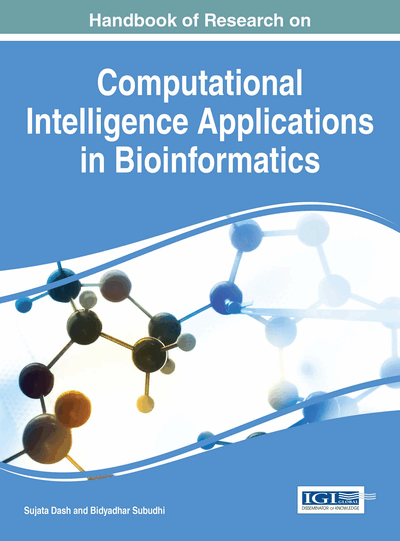# Solution of Some Differential Equation in Fuzzy Environment by Extension Principle Method and Its Application in Biomathematics

Sankar Prasad Mondal (National Institute of Technology, India)
DOI: 10.4018/978-1-5225-0427-6.ch017
Available
\$37.50
No Current Special Offers

## Abstract

The concept of fuzzy differential equations is very important for new developments of model in various fields of science and engineering problems in uncertain environments because this theory represent a natural way to modeling dynamical system under uncertain environment. In this way we can modeled mathematical biology problem associated with differential equation in fuzzy environment and solved them. In this chapter we solve two mathematical biology models which are taken in fuzzy environment. A one species prey predator model is considered with fuzzy initial data. Whereas an insect population model are described with fuzzy initial value. The solution procedures of the fuzzy differential equation are taken as extension principle method.
Chapter Preview
Top

## 1. Introduction

### 1.1 Fuzzy Differential Equation

The topic “fuzzy differential equation” (FDE) has been speedily developing in recent years. The appliance of fuzzy differential equations is an inherent way to model dynamic systems under possibilistic uncertainty (Zadeh (2005)). The concept of the fuzzy derivative was first initiated by Chang and Zadeh (1972). It was followed up by Dubois and Prade (1982). Other methods have been smeared by Puri and Ralescu (1983) and Goetschel and Voxman (1986). The concept of differential equations in a fuzzy environment was first formulated by Kaleva (1987). In fuzzy differential equation all derivative is deliberated as either Hukuhara or generalized derivatives. The Hukuhara differentiability has a deficiency (Bede et al., 2005; Diamond et al., 1994). The solution turns fuzzier as time goes by. Bede (2006) exhibited that a large class of BVPs has no solution if the Hukuhara derivative is applied. To exceeds this difficulty, the concept of a generalized derivative was developed (Bede & Gal, 2005; Cano & Flores, 2008) and fuzzy differential equations were smeared using this concept (Bede & Rudas, 2007; Cano et al., 2007; Cano et al., 2008; Stefanini & Bede, 2009). Khastan and Nieto (2010) set up the solutions for a large enough class of boundary value problems using the generalized derivative. Obviously the disadvantage of strongly generalized differentiability of a function in comparison H-differentiability is that, a fuzzy differential equation has no unique solution (Bede & Gal, 2005). Recently, Stefanini and Bede (2008) by the concept of generalization of the Hukuhara difference for compact convex set, introduced generalized Hukuhara differentiability (Stefanini & Bede, 2009) for fuzzy valued function and they displayed that, this concept of differentiability has relationships with weakly generalized differentiability and strongly generalized differentiability.

There are many approaches for solving FDE. Some researchers transform the FDE into equivalent fuzzy integral equation and then solve this (Allahviranloo et al., 2011; Chen et al., 2008; Regan et al., 2003). Another one is Zadeh extension principle method. In this method first solve the associated ODE and lastly fuzzify the solution and check whether it is satisfied or not. For details see Buckley and Feuring (2000, 2001). In the third approach, the fuzzy problem is converted to a crisp problem. Hüllermeier (1997), uses the concept of differential inclusion. In this way, by taking an α-cut of the initial value and the solution, the given differential equation is converted to a differential inclusion and the solution is accepted as the α-cut of the fuzzy solution. Laplace transform method is use many where in linear FDE (Allahviranloo & Ahmadi, 2010; Tolouti & Ahmadi, 2010). Recently, Mondal and Roy (2013) solve the first order Linear FDE by Lagrange multiplier method. Using generalized Hukuhara differentiability concept we transform the given FDE into two ODEs. And this ODEs also a differential equation involving the parametric form of a fuzzy number.

## Key Terms in this Chapter

Fuzzy Difference: The fuzzy number is not like crisp number. So the fuzzy difference is not same as crisp difference.

Bio-Mathematical Modeling: Modeling natural phenomena by mathematics.

Fuzzy Sets: A set which is a collection of object with graded membership function.

Bio-Mathematical Modeling with Uncertainty: Uncertainty came in bio mathematical model.

Fuzzy Derivative: Since fuzzy difference are different than crisp difference so fuzzy derivative are different

Fuzzy Differential Equation: The differential equation associated with fuzzy sets theory.

Extension Principle: A rule for operating fuzzy operation.

## Complete Chapter List

Search this Book:
Reset